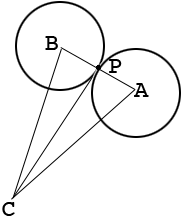SEARCH HOMEMath Central Quandaries & QueriesQuestion from Domenick: How to calculate the circumscribed and inscribed circles formed by seven .019685 diameter circles arranged in a circle with all seven circles tangent to each other?Hi Domenick,

I drew two of the seven circles with centers $A$ and $B.$ $C$ is the center of the inscribed and circumscribed circles and $P$ is the point of tangency of the two circles.By the symmetry of the diagram with all 7 circles, the measure of the angle $BCA$ is 360/7 degrees. Triangles $APC$ and $PBC$ are congruent, can you see why? Thus angle $APC$ is a right angle. You know that $|AP| = 0.019685$ units. What is the length of the line segment $CA?$

Penny* Registered trade mark of Imperial Oil Limited. Used under license.Math Central is supported by the University of Regina and the Imperial Oil Foundation.